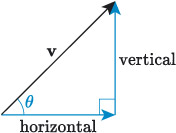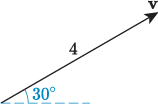Science, Maths & Technology

### Become an OU studentIntroducing vectors for engineering applications

Start this free course now. Just create an account and sign in. Enrol and complete the course for a free statement of participation or digital badge if available.

# 2.1 Horizontal and vertical componentsFigure 13 A vector and its components

Vectors are intuitively described using magnitude and direction, but they are more usefully described according to horizontal and vertical components, as illustrated in Figure 13.

A vector that can be described by a magnitude and a direction can also be described by component vectors with:

• the horizontal component pointing in the direction of the positive -axis
• the vertical component pointing in the direction of the positive -axis.

Together, a vector and its component vectors form a right-angled triangle, and the magnitudes of the three vectors define the lengths of the edges of the triangle. So, referring to Figure 13, we can use the sine and cosine functions to determine the magnitudes of the component vectors. The vector has magnitude and direction , and from these its components are calculated as follows.

The sine function is defined as

and by recognising that the vertical component is opposite the angle , we get

Similarly, the cosine function is defined as

and by recognising that the horizontal component is adjacent to the angle , we get

## Activity 7

The vector has a magnitude of 4 makes an angle of 30° with the positive -axis, as shown in the following diagram. Identify the magnitudes of the horizontal and vertical components of .